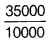Calculate the net acceleration force, the acceleration of the train and the force of wagon 1 on wagon 2

A 8000 kg engine pulls a train of 5 wagons, each of 2000 kg along a horizontal track. If the engine exerts a force of 40000 N and the track offers a friction force of 5000 N, then calculate

1. the net acceleration force,
2. the acceleration of the train and
3. the force of wagon 1 on wagon 2.
1. Net accelerating force = Force exerted by engine - Friction force
[Here, frictional force is subtracted because it opposes the motion.]
= 40000 - 5000 = 35000 = 3.5 x 1010N
2. From Newton’s second law of motion,
Accelerating force = Mass of the train x Acceleration of rain
⇒ a =Mass of train = 5 x Mass of one wagon = 5 x 2000 = 10000 kg
∴ Acceleration == 3.5 m/s2
3. Force of wagon 1 on wagon 2 = ( 35000 - 3.5 x 2000 )N = 28000N